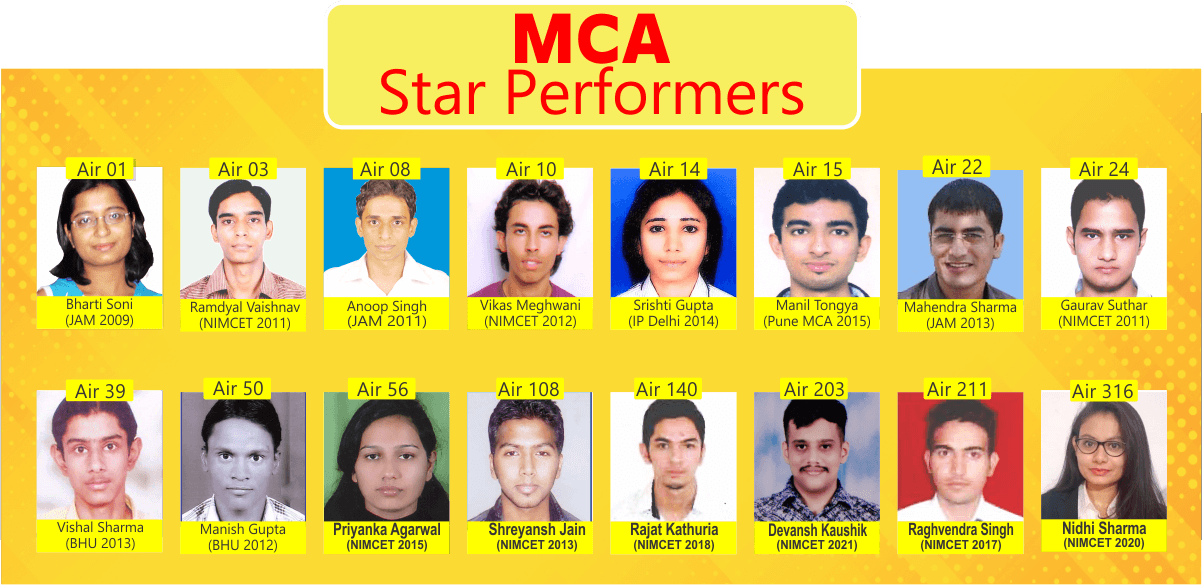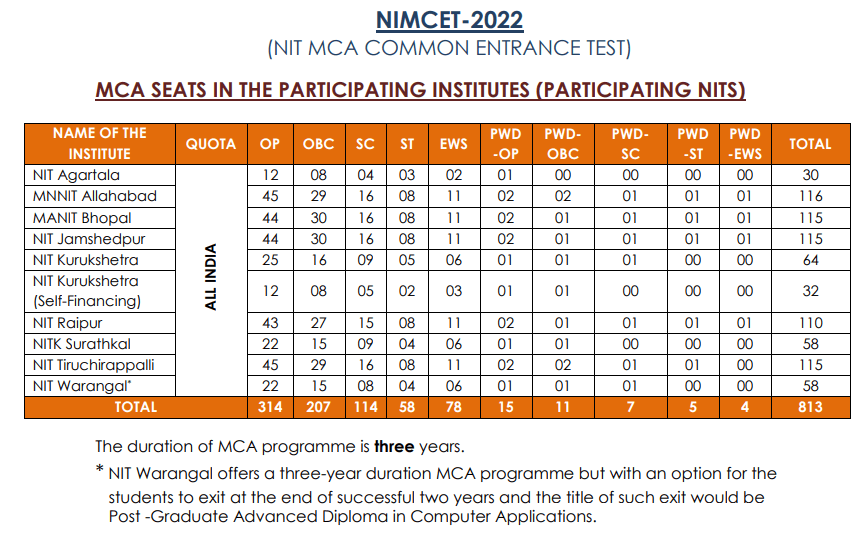## NIMCET

### NIMCET COURSE

NIMCET, The NIT MCA Common Entrance Test is a National Level Test conducted by NITs for admission to their Masterof Computer Applications (MCA) programme. The admission to the MCA programme to the nine NITs at Agartala, Allahabad, Bhopal, Jamshedpur, Kurukshetra, Raipur, Surathkal, Tiruchirappalli (Trichy), and Warangal.

####• NIMCET, The NIT MCA Common Entrance Test is a National Level Test conducted yearly by NITs. MCA from NITs is a 3 years course.

• Test consits of 120 questions which are to be solved in 120 minutes.

• There are 4 sections: Higher Maths, Reasoning, English and Computers.

• The weightage of the sections are 600, 240, 80 and 80 marks.

####• Best Faculty team in Jaipur; IIT, DU alumni, CAT 99.6%iler.

• Regular Classes on Higher Maths, Reasoning, English and Computers.

• Graded and updated study material in printed booklets.

• 30 Online Mock NIMCETs along with topic wise tests.

• Regular Doubt Solving Sessions and One to One mentoring.

####• We use LMS for online support, the complete course of NIMCET can be accessed online.

• Students get recorded videos, Practice Tests Questions and chapter wise online quizzes.

• Topic wise Previous Year Questions are available to students.

• MCA Past Papers and Solutions are available online with answer keys and detailed solutions.

## Join NIMCET 23-24 course

#### NIMCET:

NIMCET, The NIT MCA Common Entrance Test is a National Level Test conducted by NITs for admission to their Masterof Computer Applications (MCA) programme. The admission to the MCA programme to the nine NITs at Agartala, Allahabad, Bhopal, Jamshedpur, Kurukshetra, Raipur, Surathkal, Tiruchirappalli (Trichy), and Warangal.

#### Duration of MCA Course:

NITs offer a three-year duration MCA programme

#### Exam Pattern:

NIMCET test will be conducted with only one question paper containing 120 multiple choice questions covering the following subjects:

Mathematics: 50 questions
Analytical Ability & Logical Reasoning: 40 questions
Computer Awareness:  10 questions
General English: 20 questions

In the final score the weightage of Higher Mathematics, Reasoning, Computers and English questions are 12, 6, 8, 4. That makes the total marks in the paper as 50×12 + 40×6 + 10×8 + 20×4 = 1000. Students must give adequate time for practicing higher mathematics as it constitutes 60% of the total marks.

Ranking will be based on total weighted marks obtained by a candidate.

In case of a tie, it will be resolved based on weighted marks in Mathematics, then on weighted marks in Analytical Ability & Logical reasoning and then on weighted marks in Computer Awareness.

In case the tie is not resolved by the criteria stated in the above paragraph, then it will be resolved by age i.e., in favour of older candidate.#### DETAILED SYLLABUS :

MATHEMATICS :

Set Theory: Concepts of sets – Union – Intersection – Cardinality – Elementary counting permutations and combinations

Probability and Statistics: Basic concepts of probability theory, Averages, Dependent and independent events, frequency distributions, measures of central tendencies and dispersions.

Algebra: Fundamental operations in Algebra, Expansions, Factorization, simultaneous linear/quadratic equations, indices, logarithms, arithmetic, geometric and harmonic progressions, determinants and matrices.

Coordinate Geometry: Rectangular Cartesian coordinates, distance formulae, equation of a line, intersection of lines, pair of straight lines, equations of a circle, parabola, ellipse, and hyperbola.

Calculus: Limit of functions, Continuous function, Differentiation of function, tangents and normals, simple examples of maxima and minima. Integration of function by parts, by substitution and by partial fraction; Definite integrals, Applications of Definite Integrals to areas.

Vectors: Position vector, addition and subtraction of vectors, scalar and vector products and their applications to simple geometrical problems and mechanics.

Trigonometry: Simple identities, trigonometric equations, properties of triangles, solution of triangles, heights and distances, General solutions of trigonometric equations.

ANALYTICAL ABILITY AND LOGICAL REASONING :

The questions in this section will cover logical reasoning and quantitative aptitude. Some of the questions will be on comprehension of a logical situation and questions based on the facts given in the passage.

COMPUTER AWARENESS :

Computer Basics: Organization of a Computer, Central Processing Unit (CPU), Structure of instructions in CPU, input/output devices, computer memory, back-up devices. Representation of characters, integers and fractions, binary and hexadecimal representations, Binary Arithmetic: Addition, subtraction, multiplication, division, simple arithmetic and two’s complement arithmetic, floating point representation of numbers, Boolean algebra, truth tables, Venn diagrams.

GENERAL ENGLISH:

Questions in this section will be designed to test the candidates’ general understanding of the English language. There will be questions on the following topics.

Comprehension, Vocabulary, Basic English Grammar (like usage of correct forms of verbs, prepositions and articles), Word power, Synonyms and Antonyms, Meanings of words and phrases, Technical writing.

#### Number of seats:

Here is the number of seats in participating NITS.#### Participating INSTITUTES:

NIT Agartala, MNNIT Allahabad, MANIT Bhopal, MNIT Suratkal, NIT Jamshedpur, NIT Kurukshetra, NIT Raipur, NIT Trichy, NIT Warangal

For further details:

https://nimcet.in/